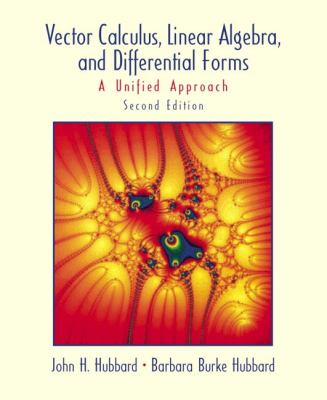164835

9780130414083

# Vector Calculus, Linear Algebra, and Differential Forms A Unified Approach\$95.85
\$3.95 Shipping
List Price
\$105.33
Discount
9% Off
You Save
\$9.48

• Condition: Good
• Provider: seattlegoodwill Contact
• Provider Rating:
95%
• Ships From: Seattle, WA
• Shipping: Standard
• Comments: May have some shelf-wear due to normal use.30-day money back guarantee

Most renters respond to questions in 48 hours or less.
The response will be emailed to you.
• ISBN-13: 9780130414083
• ISBN: 0130414085
• Edition: 2
• Publication Date: 2001
• Publisher: Prentice Hall PTR

### AUTHOR

Hubbard, John H., Hubbard, Barbara Burke

### SUMMARY

... The numerical interpretation ... is however necessary . ... So long as it is not obtained, the solutions may be said to remain incomplete and useless, and the truth which it is proposed to discover is no less hidden in the formulae of analysis than it was in the physical problem itself. - Joseph Fourier,The Analytic Theory of Heat Chapters 1 through 6 of this book cover most of the standard topics in multivariate calculus and a substantial part of a standard first course in linear algebra. If, in addition, one teaches the proofs in Appendix A, the book can be used as a textbook for a course in analysis. The organization and selection of material differs from the standard approach in three ways. First, we believe that at this level linear algebra should be more a convenient setting and language for multivariate calculus than a subject in its own right. The guiding principle of this unified approach is that locally, a nonlinear function behaves like its derivative. Thus whenever we have a question about a nonlinear function we will answer it by looking carefully at a linear transformation: its derivative. In this approach, everything learned about linear algebra pays off twice: first for understanding linear equations, then as a tool for understanding nonlinear equations. We discuss abstract vector spaces in Section 2.6, but the emphasis is on R', as we believe that most students find it easiest to move from the concrete to the abstract. Second, we emphasize computationally effective algorithms, and prove theorems by showing that those algorithms really work. We feel this better reflects the way this mathematics is used today, in both applied and in pure mathematics. Moreover, it can be done with no loss of rigor. For linear equations, row reduction (the practical algorithm) is the central tool from which everything else follows, and we use row reduction to prove all the standard results about dimension and rank. For nonlinear equations, the cornerstone is Newton's method, the best and most widely used method for solving nonlinear equations; we use it both as a computational tool and in proving the inverse and implicit function theorems. We include a section on numerical methods of integration, and we encourage the use of computers both to reduce tedious calculations and as an aid in visualizing curves and surfaces.Third, we use differential forms to generalize the fundamental theorem of calculus to higher dimensions. The great conceptual simplifications gained by doing electromagnetism in the language of forms is a central motivation for using forms, and we will apply the language of forms to electromagnetism in a subsequent volume. In our experience, differential forms can be taught to freshmen and sophomores, if forms are presented geometrically, as integrands that take an oriented piece of a curve, surface, or manifold, and return a number. We are aware that students taking courses in other fields need to master the language of vector calculus, and we devote three sections of Chapter 6 to integrating the standard vector calculus into the language of forms. Chapter 0 is intended as a resource. Students should not feel that they need to read it before beginning Chapter 1. Another resource is the inside back cover, which lists some useful formulas. Numbering of theorems, examples, and equations Theorems, lemmas, propositions, corollaries, and examples share the same numbering system: Proposition 2.3.8 is not the eighth proposition of Section 2.3; it is the eighth numbered item of that section. We often refer back to theorems, examples, and so on, and hope this numbering will make them easier to find. Figures and tables share their own numbering system; Figure 4.5.2 is the second figure or table of Section 4.5. Virtually all displayed equations are numbered, with the numbers given at right; Equation 4Hubbard, John H. is the author of 'Vector Calculus, Linear Algebra, and Differential Forms A Unified Approach', published 2001 under ISBN 9780130414083 and ISBN 0130414085.

You can find lots of answers to common customer questions in our FAQs

View a detailed breakdown of our shipping prices